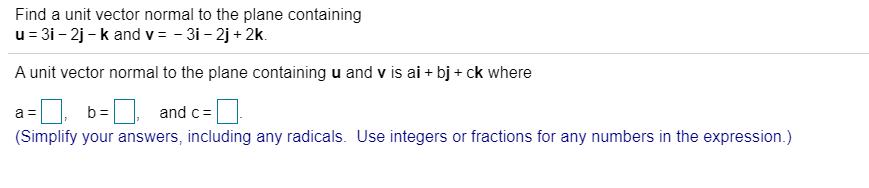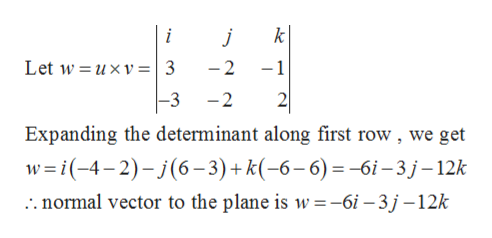# Find a unit vector normal to the plane containingu = 31 - 2j - k and v = - 3i - 2j + 2k.A unit vector normal to the plane containing u and v is ai + bj + ck whereb =and c=(Simplify your answers, including any radicals. Use integers or fractions for any numbers in the expression.)

Question
36 viewshelp_outlineImage TranscriptioncloseFind a unit vector normal to the plane containing u = 31 - 2j - k and v = - 3i - 2j + 2k. A unit vector normal to the plane containing u and v is ai + bj + ck where b = and c= (Simplify your answers, including any radicals. Use integers or fractions for any numbers in the expression.) fullscreen
check_circle

Step 1

Given, the plane containing the vectors

Step 2

Now the unit normal (perpendicular) vector to the given pl...help_outlineImage Transcriptionclosek i Let w = uxv=| 3 -1 -2 2 |-3 -2 Expanding the determinant along first row , we get w =i(-4-2)- j(6– 3)+ k(-6–6)=-6i– 3 j–12k .. normal vector to the plane is w=-6i – 3 j –12k fullscreen

### Want to see the full answer?

See Solution

#### Want to see this answer and more?

Solutions are written by subject experts who are available 24/7. Questions are typically answered within 1 hour.*

See Solution
*Response times may vary by subject and question.
Tagged in

### Trigonometry